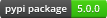django-fractions¶Fraction display and form fields for Django

Documentation¶

The full documentation is at https://django-fractions.readthedocs.org.

Quickstart¶

Install django-fractions:

pip install django-fractions

Then use it in a project:

import djfractions

In templates:

{% display_fraction 1.25 %}

In Forms:

from djfractions.forms import DecimalFractionField
from django import forms

class MyForm(forms.Form):
a_fraction = DecimalFractionField()

Features¶

• Template tag for displaying float and Decimal values as fractions including mixed numbers
• DecimalFractionField form field which handles input such as “1/4”, “1 1/2”, “1 and 1/2”, and converts to a decimal.Decimal instance

TODO¶

• Add unicode_fraction template tag to display the unicode fraction entity if available
• forms.FloatDecimalField to return a float rather than Decimal
• forms.SplitFractionWidget for having separate numerator and denominator form fields
• forms.SplitMixedFractionWidget for handling mixed number fractions with separate fields
• models.DecimalBackedFractionField() to store a Decimal value but return/accept it as a fraction
• models.FloatBackedFractionField() to store a Decimal value but return/accept it as a fraction